# Sample Size Calculator: Two Parallel-Sample Proportions

Hypothesis: Two-Sided Equality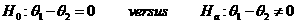Data Input: (Help) (Example)

 Input Results α β n1 θ1 n2 θ2 N r

Note:

 Variables Descriptions α Two-sided significance level 1-β Power of the test θ1 Expected success proportions of sample one θ2 Expected success proportions of sample two r Ratio of sample size of group two to group one n1 Sample size of sample one n2 Sample size of sample two N Total sample size

Help Aids

Application: To test whether there is a difference between the mean response rates of the test drug and the reference drug; the following hypotheses are usually used:Procedure:

1. Enter

a)      value of α, the probability of type I error

b)      value of β, the probability of type II error

c)      value of θ1, a true mean response rate of a test drug

d)     value of θ2, the true mean response rates of a control drug

e)      value of r, allocation ratio,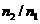.

1. Click the button “Calculate” to obtain result sample size of each group n.

Formula: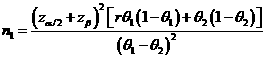(*)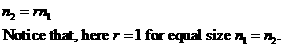Notations:

α:               The probability of type I error (significance level) is the probability of rejecting the true null hypothesis.

β:               The probability of type II error (1 – power of the test) is the probability of failing to reject the false null hypothesis.

θ12:         The difference between the true mean response rates of a group1 (i.e., a test drug (θ1) and group2 (i.e., a control (θ2))).

r:                The allocation ratio,. i.e., r=1 for equal allocation.

Examples

Example 1: Suppose that a difference of θ1–θ2=20% in clinical response of cure is considered of clinically meaningful difference between the two anti-microbial agents. By (*), assuming that the true rate for the active control agent is 65% (θ1=0.65 and θ2=0.85), then the required sample size with equal allocation (r=1) to achieve an 80% power (β=0.2) at α=0.05 can be determined by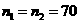.

Reference:

1. Casagrande, Pike and Smith (1978), Biometrics 34: 483-486.
2. Chow, Shao and Wang, Sample Size Calculations In Clinical Research, Taylor & Francis, NY. (2003) Page 87.
3. Flesis J.L., Statistical Methods for Rates and Proportions (2nd edition). Wiley: New York, 1981.

Top